# Value Rule

Follow

The Value rule performs a simple calculation using values from other fields in the document.

Example 1:  Calculate Extended Price1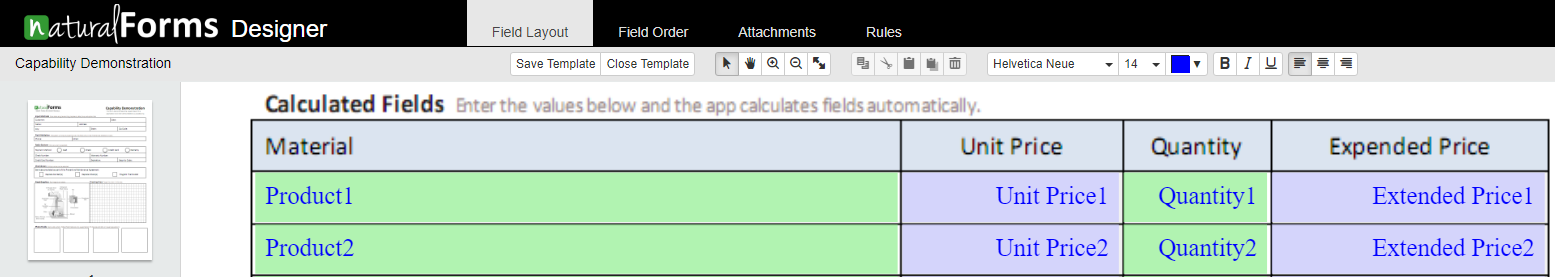Select the field you wish to apply the rule to.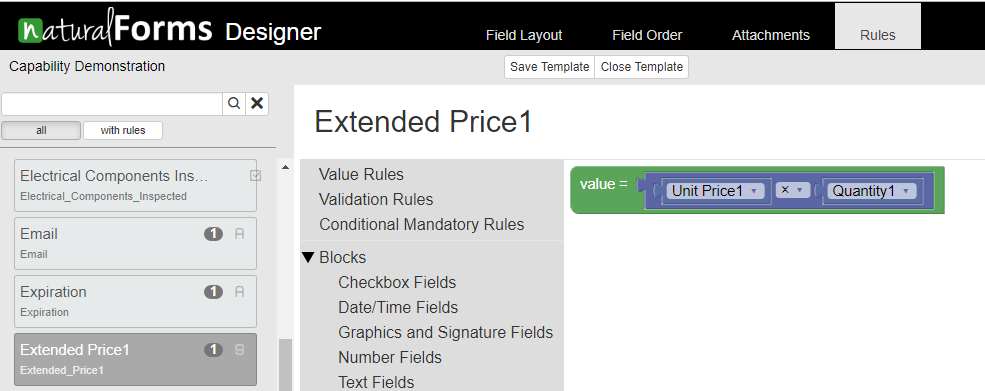You will then Select Value Rules.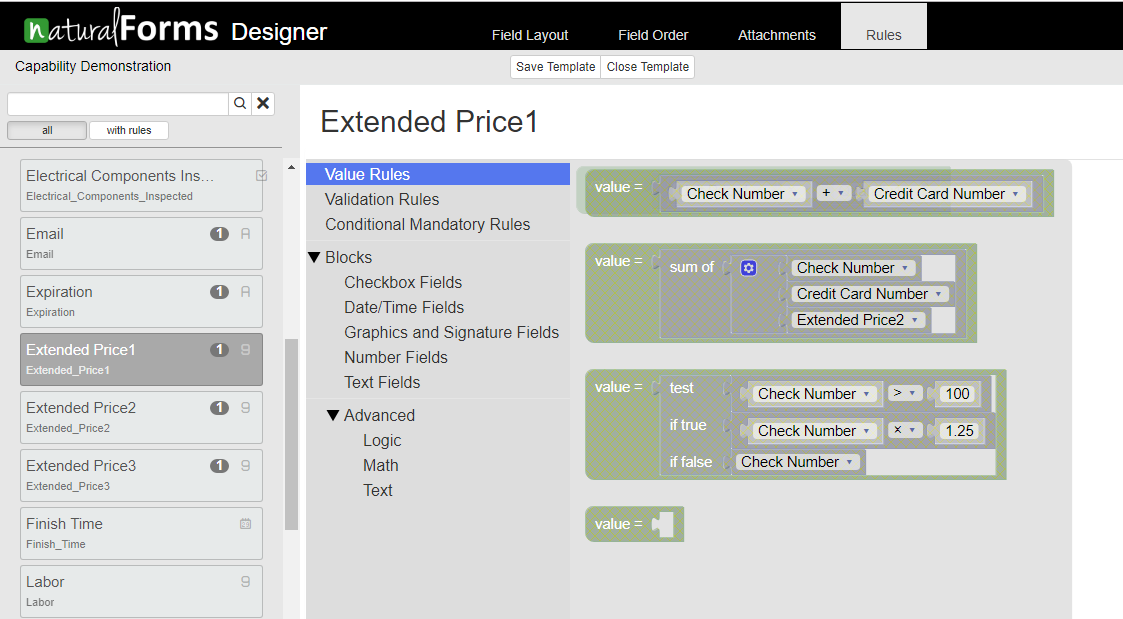Select the type of value rule that you want to use, we will use the Basic Math Function.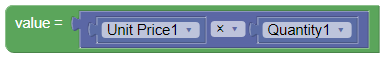Choose the fields that need to be multiplied: Unit Price 1 and Quantity1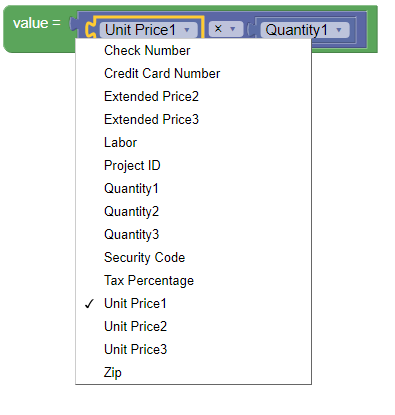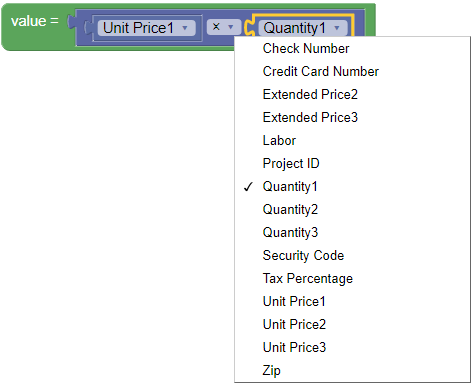Select the mathematical function needed: Multiplication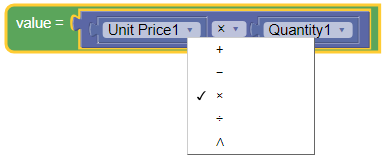Every time the Unit Price1 or the Quantity1 fields are updated, naturalForms will re-calculate the The Total will be updated every time that either subtotals, paid amount, or tax changes in naturalForms.

Here is what your rule should look like: